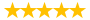10% Discount

# Assignment_Design of experiment analysis

Question # 00712178 Posted By: Sushmitha Updated on: 10/23/2018 01:02 PM Due on: 10/05/2018
Subject Statistics Topic General Statistics Tutorials:
Question

SHORT ANSWER.  Write the word or phrase that best completes each statement or answers the question. Make sure CLARIFY/JUSTIFYyour answers with concise phrase or word

1) A large laboratory has four types of devices used to determine the pH of soil samples. Thelaboratory wants to determine whether there are differences in the average readings given bythese devices. The lab uses 24 soil samples having known pH in the study and randomly assignssix of the samples to each device. The soil samples are tested, and the response recorded for eachsample is the difference between the pH reading of the device and the known pH of the soil.These values, along with summary statistics, are given in the following table.

1. Based on your intuition, is there evidence to indicate any difference among the mean differences in pH readings for the four devices?
2. Run an analysis of variance to confirm or reject your conclusion in part (a). use a α=.05.
3. Compute the p-value of the F test in part (b).
4. What conditions must be satisfied for your analysis in parts (b) and (c) to be valid?
5. Suppose the 24 soil samples have widely different pH values. What problems may occur by simply randomly assigning the soil samples to the different devices?

2) A computer ANOVA output is shown below. Fill in the blanks. Please may give bounds on the P-value.Provide your calculation details.

 One-way ANOVA Source    DF       SS    MS    F    P Factor     3    36.15   ?    ?    ? Error      ?        ?    ? Total     19   196.04

3)The tensile strength of Portland cement is being studied. Four different mixing techniques can be used economically. A completely randomized experiment was conducted and the following data were collected:

 Mixing Technique Tensile Strength (lb/in2) 1 3129 3000 2865 2890 2 3200 3300 2975 3150 3 2800 2900 2985 3050 4 2600 2700 2600 2765
1. Test the hypothesis that mixing techniques affect the strength of the cement. Use α=0.05.Complete the calculation manually.
2. Construct boxplots display to compare the mean tensile strengths for the four mixing techniques. What are your conclusions?
3. Construct a normal probability plot. What conclusion would you draw about the validity of the normality assumption?

4) Given the sample data below, test the claim that the populations have the same mean. Use a significance level of 0.05.Complete the calculation manually.

5) Random samples of four different models of cars were selected and the gas mileage of each car was measured. The results are shown below.

Test the claim that the four different models have the same population mean. Use a significance level of 0.05.Complete the calculation manually.

6) Use the data given below to verify that the t test for independent samples and the ANOVA method are equivalent.

i) Use a t test with a 0.05 significance level to test the claim that the two samples come from populations with the same means.

ii) Use the ANOVA method with a 0.05 significance level to test the same claim.

iii) Verify that the squares of the t test statistic and the critical value are equal to the F test statistic and critical value.

Attachments
Tutorials for this Question
1. 10% Discount

## Solution: A large laboratory has four types of devices used to determine

Tutorial # 00712398 Posted By: neil2103 Posted on: 10/23/2018 01:29 PM
Puchased By: 3
Tutorial Preview
The solution of A large laboratory has four types of devices used to determine...
Attachments
Homework_5_DOE_(1).docx (321.21 KB)
Recent Feedback
Rated By Feedback Comments Rated On
Su...thaHighly satisfactory results 11/23/2018

Great! We have found the solution of this question!